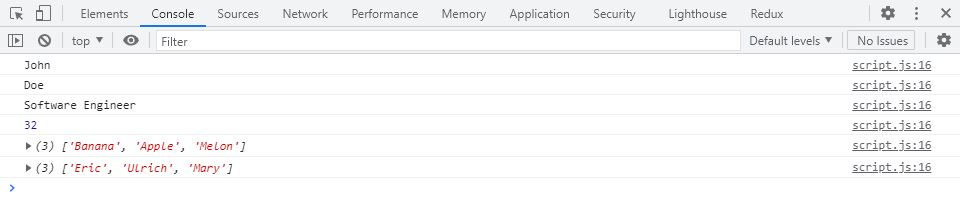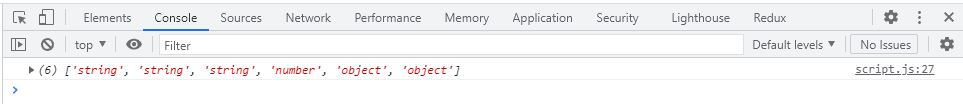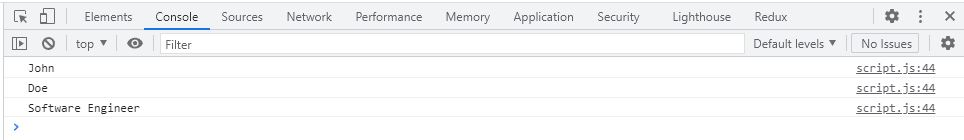# const - JS | lectureJavaScript Fundamentals 2

Looping Arrays, Break & Continue

In this lecture, we will explore some more features of the for loop with some examples. One of the most used applications of the for loop is to loop through arrays.

Let's consider the user array we used in the intro to object lecture.

script.js
``````const user = [
'John',
'Doe',
'Software Engineer',
32,
['Banana', 'Apple', 'Melon'],
['Eric', 'Ulrich', 'Mary'],
];``````

We can now use the for loop to loop over this array. For now, let's say we want to log each element of the array to the console.

script.js
``````/**
* I used i because that is a traditional counter variable name.
*
* Our counter needs to start at zero because if you remember an
* array is zero based when it comes to reading elements out of an
* array.
*
* i++ because as you already know, is to update the counter by 1
* since we want to read each element of the array.
*/
for (let i = 0; i < user.length; i++) {
console.log(user[i]);
}``````Up till now, nothing complicated. Let's now say we want to create an array that contains the type of each element of our `user` array. You may see these examples as useless or boring, and I agree, but the main idea here is to show you how the different features of the language work.

script.js
``````// Assigning the variable arrayTypes an empty array
const arrayTypes = [];

for (let i = 0; i < user.length; i++) {
// This is just the same as doing arrayTypes = 'string'
arrayTypes[i] = typeof user[i];
}

console.log(arrayTypes);``````We can also use the `push` method, which we saw in one of the previous lectures, to fill in the arrayTypes variable.

script.js
``````for (let i = 0; i < user.length; i++) {
// Remember that the push method adds a new element to the end of the array
arrayTypes.push(typeof user[i]);
}``````

Using the push method is much cleaner, but if you prefer the first, that's ok. What matters here is that you understand the logic of how to construct the different parts of the for loop in other to loop the array.

Let's consider another example where we have some years of birth in an array, and we want to calculate their ages and store them in another array.

``````const years = [1990, 1991, 1995, 1996];
const ages = [];

//What we have to do is to loop through the years array and fill the ages array
for (let i = 0; i < years.length; i++) {
ages.push(2021 - years[i]);
}

console.log(ages); // Result ==> [31, 30, 26, 25]``````

To finish, let's learn about two important statements for loops. That is, the `continue` and `break` statement. The `continue` statement is used to exit the current iteration of the loop and continue to the next one. On the other hand, `break` is used to terminate the whole loop completely.

Let's say, for example, that we only want to log elements in our user array that are strings. For this, we can use the continue statement because, with continue, we can exit the current iteration of the loop.

script.js
``````for (let i = 0; i < user.length; i++) {
if (typeof user[i] !== 'string') {
continue;
}
console.log(user[i]);
}``````On executing our code, we can see that the last three elements of our array are not logged to the console because we basically skipped them. For example, we can see that the fourth element of our array (`user`) is `32`, which is a `number` type. In our `for loop` when testing the `if condition`, it will be true because `number` is different from `string`, making the code within the `if block` to be executed.

We then have the `continue` statement within the `if block`, which will exit the current iteration; thus, our console will not log the current iteration.

The `break` statement, on the other hand, as we previously said, terminates the whole loop. Let's say we want to log no other element after we find a `number`. That is, after we have found the number 32 in our array, nothing else should be printed.

script.js
``````for (let i = 0; i < user.length; i++) {
if (typeof user[i] === 'number') break;
console.log(user[i]);
}``````